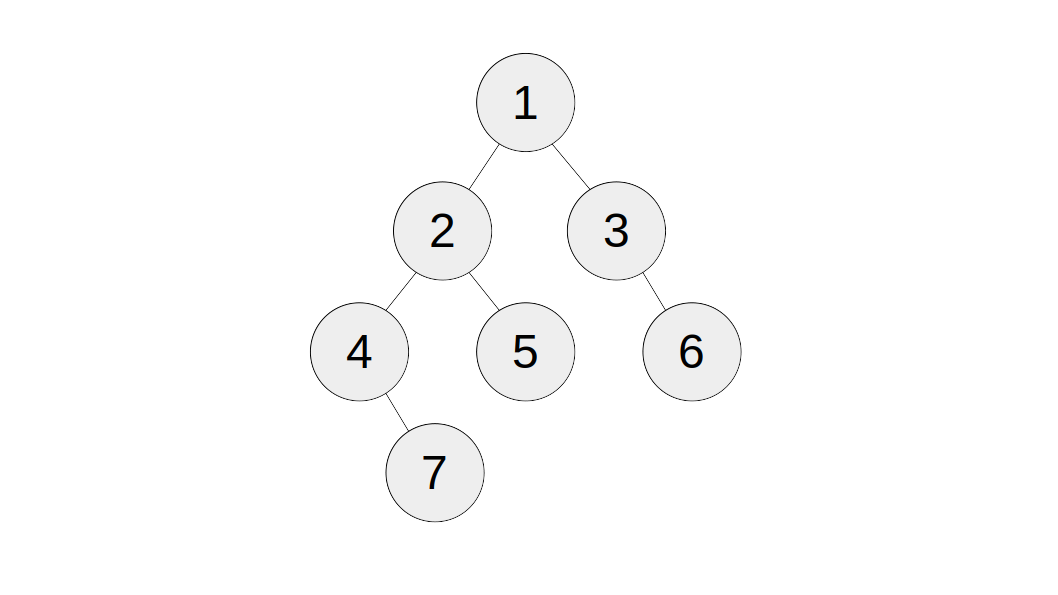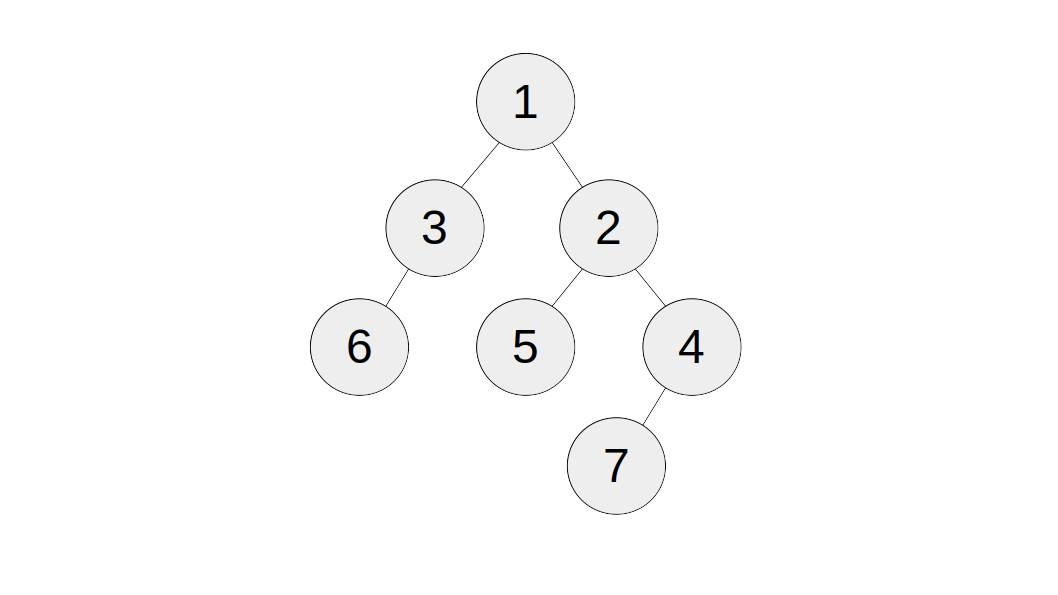# Invert Binary Tree

Given a binary tree, invert it and return the new value. You may invert it in-place.

To "invert" a binary tree, switch the left subtree and the right subtree, and invert them both. Inverting an empty tree does nothing.

### Input

• `tree`: a binary tree that needs to be inverted.

### Output

The inverted binary tree.

### Examples

#### Example 1:

Input:

``1tree = <See explanation>``

Output: `<See explanation>`

Explanation:

Original tree:Inverted tree: